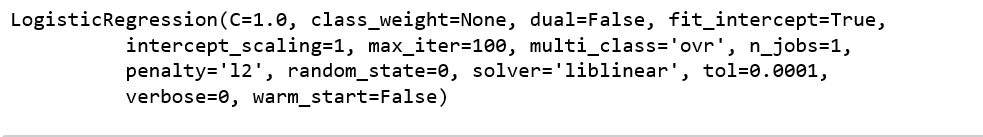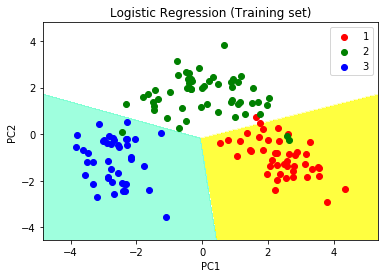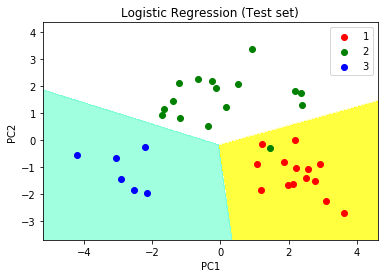# Principal Component Analysis with Python

Principal Component Analyis is basically a statistical procedure to convert a set of observation of possibly correlated variables into a set of values of linearly uncorrelated variables.
Each of the principal components is chosen in such a way so that it would describe most of the still available variance and all these principal components are orthogonal to each other. In all principal components first principal component has maximum variance.

Uses of PCA:

• It is used to find inter-relation between variables in the data.
• It is used to interpret and visualize data.
• As number of variables are decreasing it makes further analysis simpler.
• It’s often used to visualize genetic distance and relatedness between populations.

These are basically performed on square symmetric matrix. It can be a pure sums of squares and cross products matrix or Covariance matrix or Correlation matrix. A correlation matrix is used if the individual variance differs much.

Objectives of PCA:

• It is basically a non-dependent procedure in which it reduces attribute space from a large number of variables to a smaller number of factors.
• PCA is basically a dimension reduction process but there is no guarantee that the dimension is interpretable.
• Main task in this PCA is to select a subset of variables from a larger set, based on which original variables have the highest correlation with the principal amount.

Principal Axis Method: PCA basically search a linear combination of variables so that we can extract maximum variance from the variables. Once this process completes it removes it and search for another linear combination which gives an explanation about the maximum proportion of remaining variance which basically leads to orthogonal factors. In this method, we analyze total variance.

Eigenvector: It is a non-zero vector that stays parallel after matrix multiplication. Let’s suppose x is eigen vector of dimension r of matrix M with dimension r*r if Mx and x are parallel. Then we need to solve Mx=Ax where both x and A are unknown to get eigen vector and eigen values.
Under Eigen-Vectors we can say that Principal components show both common and unique variance of the variable. Basically, it is variance focused approach seeking to reproduce total variance and correlation with all components. The principal components are basically the linear combinations of the original variables weighted by their contribution to explain the variance in a particular orthogonal dimension.

Eigen Values: It is basically known as characteristic roots. It basically measures the variance in all variables which is accounted for by that factor. The ratio of eigenvalues is the ratio of explanatory importance of the factors with respect to the variables. If the factor is low then it is contributing less in explanation of variables. In simple words, it measures the amount of variance in the total given database accounted by the factor. We can calculate the factor’s eigen value as the sum of its squared factor loading for all the variables.

Now, Let’s understand Principal Component Analysis with Python.

Step 1: Importing the libraries

 `# importing required libraries ` `import` `numpy as np ` `import` `matplotlib.pyplot as plt ` `import` `pandas as pd `

Step 2: Importing the data set

Import the dataset and distributing the dataset into X and y components for data analysis.

 `# importing or loading the dataset ` `dataset ``=` `pd.read_csv(``'wines.csv'``) ` ` `  `# distributing the dataset into two components X and Y ` `X ``=` `dataset.iloc[:, ``0``:``13``].values ` `y ``=` `dataset.iloc[:, ``13``].values `

Step 3: Splitting the dataset into the Training set and Test set

 `# Splitting the X and Y into the ` `# Training set and Testing set ` `from` `sklearn.model_selection ``import` `train_test_split ` ` `  `X_train, X_test, y_train, y_test ``=` `train_test_split(X, y, test_size ``=` `0.2``, random_state ``=` `0``) `

Step 4: Feature Scaling

Doing the pre-processing part on training and testing set such as fitting the Standard scale.

 `# performing preprocessing part ` `from` `sklearn.preprocessing ``import` `StandardScaler ` `sc ``=` `StandardScaler() ` ` `  `X_train ``=` `sc.fit_transform(X_train) ` `X_test ``=` `sc.transform(X_test) `

Step 5: Applying PCA function

Applying the PCA function into training and testing set for analysis.

 `# Applying PCA function on training ` `# and testing set of X component ` `from` `sklearn.decomposition ``import` `PCA ` ` `  `pca ``=` `PCA(n_components ``=` `2``) ` ` `  `X_train ``=` `pca.fit_transform(X_train) ` `X_test ``=` `pca.transform(X_test) ` ` `  `explained_variance ``=` `pca.explained_variance_ratio_ `

Step 6: Fitting Logistic Regression To the training set

 `# Fitting Logistic Regression To the training set ` `from` `sklearn.linear_model ``import` `LogisticRegression   ` ` `  `classifier ``=` `LogisticRegression(random_state ``=` `0``) ` `classifier.fit(X_train, y_train) `Step 7: Predicting the test set result

 `# Predicting the test set result using  ` `# predict function under LogisticRegression  ` `y_pred ``=` `classifier.predict(X_test) `

Step 8: Making the confusion matrix

 `# making confusion matrix between ` `#  test set of Y and predicted value. ` `from` `sklearn.metrics ``import` `confusion_matrix ` ` `  `cm ``=` `confusion_matrix(y_test, y_pred) `

Step 9: Predicting the training set result

 `# Predicting the training set ` `# result through scatter plot  ` `from` `matplotlib.colors ``import` `ListedColormap ` ` `  `X_set, y_set ``=` `X_train, y_train ` `X1, X2 ``=` `np.meshgrid(np.arange(start ``=` `X_set[:, ``0``].``min``() ``-` `1``, ` `                     ``stop ``=` `X_set[:, ``0``].``max``() ``+` `1``, step ``=` `0.01``), ` `                     ``np.arange(start ``=` `X_set[:, ``1``].``min``() ``-` `1``, ` `                     ``stop ``=` `X_set[:, ``1``].``max``() ``+` `1``, step ``=` `0.01``)) ` ` `  `plt.contourf(X1, X2, classifier.predict(np.array([X1.ravel(), ` `             ``X2.ravel()]).T).reshape(X1.shape), alpha ``=` `0.75``, ` `             ``cmap ``=` `ListedColormap((``'yellow'``, ``'white'``, ``'aquamarine'``))) ` ` `  `plt.xlim(X1.``min``(), X1.``max``()) ` `plt.ylim(X2.``min``(), X2.``max``()) ` ` `  `for` `i, j ``in` `enumerate``(np.unique(y_set)): ` `    ``plt.scatter(X_set[y_set ``=``=` `j, ``0``], X_set[y_set ``=``=` `j, ``1``], ` `                ``c ``=` `ListedColormap((``'red'``, ``'green'``, ``'blue'``))(i), label ``=` `j) ` ` `  `plt.title(``'Logistic Regression (Training set)'``) ` `plt.xlabel(``'PC1'``) ``# for Xlabel ` `plt.ylabel(``'PC2'``) ``# for Ylabel ` `plt.legend() ``# to show legend ` ` `  `# show scatter plot ` `plt.show() `Step 10: Visualising the Test set results

 `# Visualising the Test set results through scatter plot ` `from` `matplotlib.colors ``import` `ListedColormap ` ` `  `X_set, y_set ``=` `X_test, y_test ` ` `  `X1, X2 ``=` `np.meshgrid(np.arange(start ``=` `X_set[:, ``0``].``min``() ``-` `1``, ` `                     ``stop ``=` `X_set[:, ``0``].``max``() ``+` `1``, step ``=` `0.01``), ` `                     ``np.arange(start ``=` `X_set[:, ``1``].``min``() ``-` `1``, ` `                     ``stop ``=` `X_set[:, ``1``].``max``() ``+` `1``, step ``=` `0.01``)) ` ` `  `plt.contourf(X1, X2, classifier.predict(np.array([X1.ravel(), ` `             ``X2.ravel()]).T).reshape(X1.shape), alpha ``=` `0.75``, ` `             ``cmap ``=` `ListedColormap((``'yellow'``, ``'white'``, ``'aquamarine'``)))  ` ` `  `plt.xlim(X1.``min``(), X1.``max``()) ` `plt.ylim(X2.``min``(), X2.``max``()) ` ` `  `for` `i, j ``in` `enumerate``(np.unique(y_set)): ` `    ``plt.scatter(X_set[y_set ``=``=` `j, ``0``], X_set[y_set ``=``=` `j, ``1``], ` `                ``c ``=` `ListedColormap((``'red'``, ``'green'``, ``'blue'``))(i), label ``=` `j) ` ` `  `# title for scatter plot ` `plt.title(``'Logistic Regression (Test set)'``)  ` `plt.xlabel(``'PC1'``) ``# for Xlabel ` `plt.ylabel(``'PC2'``) ``# for Ylabel ` `plt.legend() ` ` `  `# show scatter plot ` `plt.show() `Attention geek! Strengthen your foundations with the Python Programming Foundation Course and learn the basics.

To begin with, your interview preparations Enhance your Data Structures concepts with the Python DS Course.

My Personal Notes arrow_drop_upCheck out this Author's contributed articles.

If you like GeeksforGeeks and would like to contribute, you can also write an article using contribute.geeksforgeeks.org or mail your article to contribute@geeksforgeeks.org. See your article appearing on the GeeksforGeeks main page and help other Geeks.

Please Improve this article if you find anything incorrect by clicking on the "Improve Article" button below.